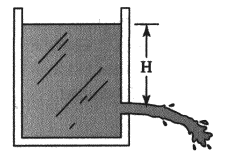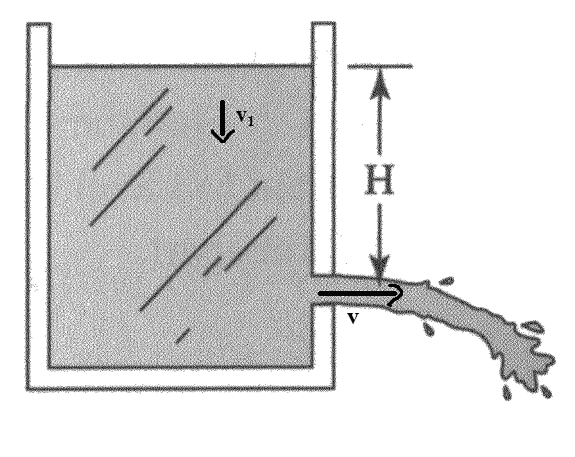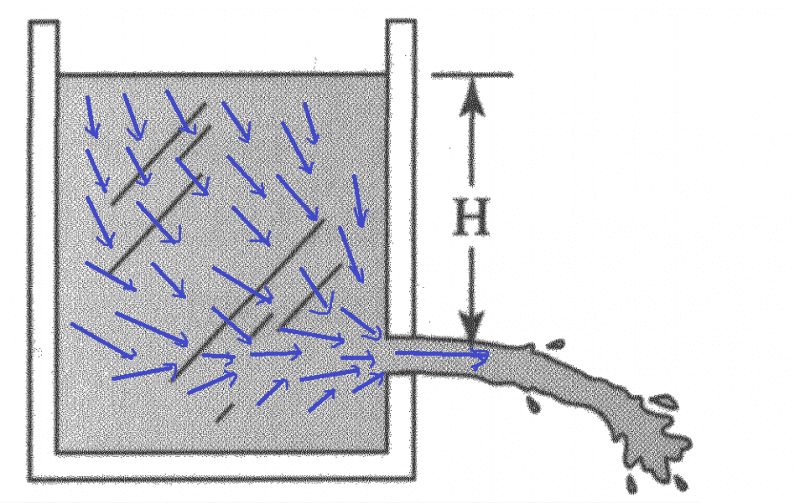# Calculating velocity for water pressure

• arpon
But, I couldn't understand how it is applied in this example.In summary, the conversation discusses a problem involving a tank of liquid with a small hole at the bottom. The question asks for the speed at which the liquid will emerge from the hole if there is no internal friction. The conversation includes various equations and attempts at solving the problem, with one approach using force arguments and another using energy considerations. However, the correct solution involves solving the inviscid flow equations for the velocities, pressures, and streamlines approaching the outlet. The use of the Bernoulli equation is also mentioned.

## Homework StatementA tank of cross-sectional area ##A## contains a liquid having density ##\rho##. The liquid squirts freely from a small hole of area ##a## at a distance ##H## below the free surface of the liquid.
If the liquid has no internal friction(viscosity), with what speed does it emerge?

## Homework Equations

##p = H\rho g ##
##F = \frac{\Delta (mv)}{\Delta t}##

## The Attempt at a Solution

##F = pa = aH\rho g ##
Again, ## F = \frac{\Delta (mv)}{\Delta t} = v \cdot \frac{\Delta m}{\Delta t}## ; because, ##v## is constant;
Now, ## \frac{\Delta m}{\Delta t} = \frac{a (\Delta x) \rho }{\Delta t} = a \rho v ## ; because, ##\frac{\Delta x}{\Delta t} = v ##
So, ## aH\rho g = v \cdot a \rho v = a \rho v^2 ##
Finally, ## v = \sqrt{Hg}##
But, it doesn't match the answer.

Last edited:
It seems you are randomly sticking equations together. If you are going to use force arguments you need to consider what mass the force is acting on, if that mass is changing, and whether or not its velocity is actually constant as you assume.

Or you could take the easy way out and solve it using energy considerations instead.

Orodruin said:
It seems you are randomly sticking equations together. If you are going to use force arguments you need to consider what mass the force is acting on, if that mass is changing, and whether or not its velocity is actually constant as you assume.
Could not understand what is the problem in my approach.
Orodruin said:
you could take the easy way out and solve it using energy considerations instead.
OK. I calculated in this way:
##\frac {dP.E}{dt} = - \frac {d K.E}{dt} ## ; because, P.E. + K.E. = constant
##\frac {d}{dt} (mg \frac{h}{2}) = - \frac{d}{dt} (\frac{1}{2} mv^2)##; average height is ##\frac{h}{2}##
##\frac {d}{dt} (Ah\rho g \frac{h}{2}) = - \frac{1}{2} \frac{dm}{dt} v^2##; as, ##v## is constant;
##\frac {1}{2} A \rho g \frac {d}{dt} (h^2) = - \frac{1}{2} \frac{d}{dt}(- Ah \rho) v^2##
## g \cdot 2h \frac {dh}{dt} = \frac{dh}{dt} v^2##
## v = \sqrt{2gh}## ; which is the expected answer.
But still couldn't understand what's wrong in my previous approach?

arpon said:
But still couldn't understand what's wrong in my previous approach?
As I said, you need to consider what volume the force is acting on. Before you have done that and specified your other assumptions, you really cannot tell if what you are doing makes sense.

arpon said:
Could not understand what is the problem in my approach.
The problem is that you are taking it as though the water accelerates instantaneously from 0 to v. That makes it like an inelastic collision, with about half the energy being lost. In practice, a much smaller portion of the energy is lost. The water accelerates more gradually from the area surrounding the hole from inside the tank. So assume conservation of energy instead (Bernoulli's principle).
Of course, some energy is lost to turbulence, so that doesn't give exactly the right answer either. Turbulence can be reduced by fitting a Borda mouthpiece inside the tank around the hole.

To expand on what haruspex was saying, the fluid flow is converging in the region approaching the outlet hole. This is similar to what happens in a converging nozzle, where the surrounding fluid forms a sort of converging channel for the fluid approaching the hole. For an analysis of incompressible inviscid flow in a nozzle, see post #22 in the following thread: https://www.physicsforums.com/threads/fluid-question.791594/page-2#post-4973184. It has the term you are talking about for the rate of change of momentum, but it also includes the retarding force resulting from the pressure of the converging wall on the fluid. This is how the factor of 1/2 comes into play. The analysis also shows that the Bernoulli equation gives the same result as your conservation of energy analysis, and correctly yields the factor of 1/2.

Chet

Chestermiller said:
but it also includes the retarding force resulting from the pressure of the converging wall on the fluid.
But, how the retarding force acts in my example ? Would you please explain this in more detail ?

arpon said:
But, how the retarding force acts in my example ? Would you please explain this in more detail ?
Your problem is more complicated geometrically than a gradually tapering nozzle; the outer streamlines provide the converging "wall" for the inner stream flow. To solve your problem requires solving the inviscid flow equations for the velocities, pressures, and streamlines approaching the outlet. These equations are 2D PDEs. However, along any streamline, even in this more complicated case, the Bernoulli equation applies. But, to implement the bernoulli equation, you need to know the magnitude of the velocity at a given location from the solution to the PDEs.

Chet

Chestermiller said:
Your problem is more complicated geometrically than a gradually tapering nozzle; the outer streamlines provide the converging "wall" for the inner stream flow. To solve your problem requires solving the inviscid flow equations for the velocities, pressures, and streamlines approaching the outlet. These equations are 2D PDEs. However, along any streamline, even in this more complicated case, the Bernoulli equation applies. But, to implement the bernoulli equation, you need to know the magnitude of the velocity at a given location from the solution to the PDEs.
arpon said:

## Homework Statement

View attachment 78310

## The Attempt at a Solution

##F = pa = aH\rho g ##
Again, ## F = \frac{\Delta (mv)}{\Delta t} = v \cdot \frac{\Delta m}{\Delta t}## ; because, ##v## is constant;
Now, ## \frac{\Delta m}{\Delta t} = \frac{a (\Delta x) \rho }{\Delta t} = a \rho v ## ; because, ##\frac{\Delta x}{\Delta t} = v ##
So, ## aH\rho g = v \cdot a \rho v = a \rho v^2 ##
Finally, ## v = \sqrt{Hg}##
But, it doesn't match the answer.
Would you please explain what was wrong in this approach? I still cannot find out this. You said about 'retarding force' in post #6. But, I couldn't understand how it is applied in this example.

arpon said:
Would you please explain what was wrong in this approach? I still cannot find out this. You said about 'retarding force' in post #6. But, I couldn't understand how it is applied in this example.
There are a couple of things wrong with your approach, and yet you still get the correct answer to within a factor of 2.

First of all, you are assuming that all the "action" is taking place right at the exit cross section, where the pressure suddenly drops from P to atmospheric and the velocity suddenly increases from zero to the exit velocity. This would be equivalent to an impulsive force at the exit cross section (like in a collision), which is not the case. In the real physical situation, the pressure in the tank is decreasing gradually as the fluid approaches the exit cross section, and its velocity is increasing gradually. So assuming that the pressure P (far from the exit) times the cross sectional area at the exit A is the force that accelerates the fluid is incorrect.

Secondly, in the real physical situation, the fluid is not traveling in a horizontal line toward the exit. On the contrary. It is essentially converging radially toward the exit cross section, as if the exit cross section were a spherical point sink, with the surfaces of constant pressure being nearly hemispherical. Only within a distance on the order of about 1 exit cross section diameter from the exit do the streamlines straighten out and become horizontal. I hope you can see how this is very different from what you assumed.

Chet

•arpon
Probably, I have understood what you meant. Thanks for this explanation.
Chestermiller said:
But I couldn't understand this point I mentioned above.Let the velocity in the tank be ##v_1## and the velocity at the hole be ##v## ;
So, change of mass in the tank per second ##= \rho Av_1## ; where, ##A## is the cross-sectional area of the tank.
And, the amount of water flow per second at the hole is ## = \rho av## ; where, ##a## is the cross-sectional area of the hole.
So, it becomes,##\rho Av_1 = \rho av##
Hence, ## v_1 = \frac{a}{A} v ##
So, I thought , the speed of the water in the tank would be constant (which is equal to ##v_1##) and the speed of water at the hole would also be constant (## = v##). And I thought, as soon as the water reaches at the height of the hole, it suddenly changes its velocity (which is in the horizontal direction).
Would you please clarify this point ?

arpon said:
Probably, I have understood what you meant. Thanks for this explanation.

But I couldn't understand this point I mentioned above.
View attachment 78350
Let the velocity in the tank be ##v_1## and the velocity at the hole be ##v## ;
So, change of mass in the tank per second ##= \rho Av_1## ; where, ##A## is the cross-sectional area of the tank.
And, the amount of water flow per second at the hole is ## = \rho av## ; where, ##a## is the cross-sectional area of the hole.
So, it becomes,##\rho Av_1 = \rho av##
Hence, ## v_1 = \frac{a}{A} v ##
So, I thought , the speed of the water in the tank would be constant (which is equal to ##v_1##) and the speed of water at the hole would also be constant (## = v##). And I thought, as soon as the water reaches at the height of the hole, it suddenly changes its velocity (which is in the horizontal direction).
Would you please clarify this point ?
Imagine a speck of dust in the middle of the water. It won't descend vertically at a steady speed until it reaches the level of the hole, then suddenly go horizontally at exit speed. Rather, it will gradually curve around towards the exit as it approaches the hole level, getting faster as it does so. Admittedly, most of the acceleration is close to the exit, but it is not a sudden jump. Correspondingly, the pressure will reduce somewhat as it approaches the hole. This makes it hard to apply conservation of momentum.

haruspex said:
Imagine a speck of dust in the middle of the water. It won't descend vertically at a steady speed until it reaches the level of the hole, then suddenly go horizontally at exit speed. Rather, it will gradually curve around towards the exit as it approaches the hole level, getting faster as it does so. Admittedly, most of the acceleration is close to the exit, but it is not a sudden jump. Correspondingly, the pressure will reduce somewhat as it approaches the hole. This makes it hard to apply conservation of momentum.
But , the vertical component of the velocity of water in the tank, I think, will be constant , and will be ##v_1 = \frac {a}{A} v##, as I calculated in my earlier post.

arpon said:
But , the vertical component of the velocity of water in the tank, I think, will be constant , and will be ##v_1 = \frac {a}{A} v##, as I calculated in my earlier post.
I didn't say the vertical component would change (though it must eventually in order to exit horizontally). I said it would gradually acquire a horizontal component.

haruspex said:
I didn't say the vertical component would change (though it must eventually in order to exit horizontally). I said it would gradually acquire a horizontal component.
So, does the vertical component of the velocity change to 0 at the hole all at once?

arpon said:
So, does the vertical component of the velocity change to 0 at the hole all at once?
Surely not. Water flow will be smooth within the tank.

haruspex said:
Surely not. Water flow will be smooth within the tank.
OK. Then the vertical velocity at a certain height should differ from point to point. But, I have confusion where the vertical velocity will be greater, I think , it will be at the point nearer to the hole.

arpon said:
Probably, I have understood what you meant. Thanks for this explanation.

But I couldn't understand this point I mentioned above.
View attachment 78350
Let the velocity in the tank be ##v_1## and the velocity at the hole be ##v## ;
So, change of mass in the tank per second ##= \rho Av_1## ; where, ##A## is the cross-sectional area of the tank.
And, the amount of water flow per second at the hole is ## = \rho av## ; where, ##a## is the cross-sectional area of the hole.
So, it becomes,##\rho Av_1 = \rho av##
Hence, ## v_1 = \frac{a}{A} v ##
So, I thought , the speed of the water in the tank would be constant (which is equal to ##v_1##) and the speed of water at the hole would also be constant (## = v##). And I thought, as soon as the water reaches at the height of the hole, it suddenly changes its velocity (which is in the horizontal direction).
Would you please clarify this point ?
This is not what happens. To get an idea of how the parcels of fluid in the tank move, imagine a very long room full of people (from wall to wall) with an exit door on the long wall not very far from the corner. The short wall far from the exit door begins to move toward the people, and the people realizing that they might get squished begin to file toward the door. The wall moves in such a way that the number of people per unit area in the room remains constant. In the region close to the door, all the people will be converging radially toward the door. Even beyond the door, in the part of the room toward the non-moving short wall, people will be moving toward the door (with a velocity component opposite to that of the moving wall). So, aside from the people right next to the moving wall and rigid walls, all the people in the room will have velocity components both parallel and perpendicular to that of the moving wall, and some of their parallel components will even be opposite to that of the moving wall.

Chet

•arpon
Chestermiller said:
This is not what happens. To get an idea of how the parcels of fluid in the tank move, imagine a very long room full of people (from wall to wall) with an exit door on the long wall not very far from the corner. The short wall far from the exit door begins to move toward the people, and the people realizing that they might get squished begin to file toward the door. The wall moves in such a way that the number of people per unit area in the room remains constant. In the region close to the door, all the people will be converging radially toward the door. Even beyond the door, in the part of the room toward the non-moving short wall, people will be moving toward the door (with a velocity component opposite to that of the moving wall). So, aside from the people right next to the moving wall and rigid walls, all the people in the room will have velocity components both parallel and perpendicular to that of the moving wall, and some of their parallel components will even be opposite to that of the moving wall.
This was helpful to understand the matter. So, I think the velocity will be (roughly) something like the picture below:And I conclude, there is no easy way to calculate the exit velocity using the formula : force = rate of change of momentum with time.
It's better to use the energy conservation law for this problem.

•Chestermiller
arpon said:
This was helpful to understand the matter. So, I think the velocity will be (roughly) something like the picture below:
View attachment 78386
And I conclude, there is no easy way to calculate the exit velocity using the formula : force = rate of change of momentum with time.
It's better to use the energy conservation law for this problem.
Yes. Yes. Yes.

Chet

arpon said:
All I would add is that you have arrows rising from the lowest layer towards the exit, so there should be arrows down into that layer on the left hand side.

•arpon
haruspex said:
All I would add is that you have arrows rising from the lowest layer towards the exit, so there should be arrows down into that layer on the left hand side.
I agree with you.